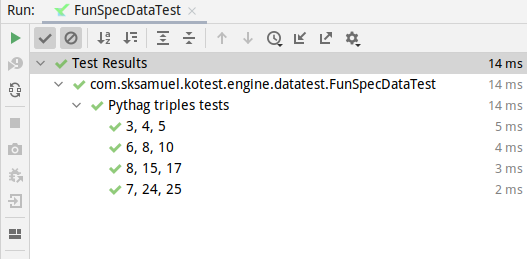# Custom Test Names

By default, the name of each test is simply the `toString()` on the input row. This typically works well for data classes.

However, we can customize this if we wish, by passing in test names into the `withData` function in the form of map, where the key is the test name, and the value is the input value for that row.

context("Pythag triples tests") {
withData(
mapOf(
"3, 4, 5" to PythagTriple(3, 4, 5),
"6, 8, 10" to PythagTriple(6, 8, 10),
"8, 15, 17" to PythagTriple(8, 15, 17),
"7, 24, 25" to PythagTriple(7, 24, 25)
)
) { (a, b, c) ->
a * a + b * b shouldBe c * c
}
}

Or we can pass a function to `withData` which take `row` as input and return the test name.

context("Pythag triples tests") {
withData(
nameFn = { t: PythagTriple -> "\${t.a}__\${t.b}__\${t.c}" },
PythagTriple(3, 4, 5),
PythagTriple(6, 8, 10),
PythagTriple(8, 15, 17),
PythagTriple(7, 24, 25)
) { (a, b, c) ->
a * a + b * b shouldBe c * c
}
}

The output from this example is now slightly clearer:## WithDataTestName#

Another alternative is to implement the `WithDataTestName` interface. When provided, the `toString()` will not be used, instead the `dataTestName` function will be invoked for each row.

data class PythagTriple(val a: Int, val b: Int, val c: Int) : WithDataTestName {
override fun dataTestName() = "\$a, \$b, \$c"
}

## Test Name Function#

Finally, another option is to provide a function directly to the `withData` method.

context("Pythag triples tests") {
withData<PythagTriple>(
{ "\${it.a}, \${it.b}, \${it.c}" },
PythagTriple(3, 4, 5),
PythagTriple(6, 8, 10),
PythagTriple(8, 15, 17),
PythagTriple(7, 24, 25)
) { (a, b, c) ->
a * a + b * b shouldBe c * c
}
}

Whether this is worth the extra effort or not depends on how readable the toString() method is on the data classes you are using.